# Foliation

on an-dimensional manifoldA decomposition of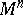into path-connected subsets, called leaves, such thatcan be covered by coordinate neighbourhoods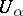with local coordinates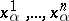, in terms of which the local leaves, that is, the connected components of the intersection of the leaves with, are given by the equations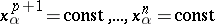. A foliation in this sense is called a topological foliation. If one also requires thathas a piecewise-linear, differentiable or analytic structure, and that the local coordinates are piecewise-linear, differentiable (of class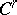) or analytic, then one obtains the definition of a piecewise-linear, differentiable (of class) or analytic foliation, respectively. The definition of a differentiable foliation of classalso makes sense when, and coincides with the definition of a topological foliation. When speaking about a differentiable foliation, it is usually understood that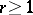. The leaves are naturally equipped with a structure of-dimensional manifolds (topological, piecewise-linear, differentiable, or analytic) and are submanifolds (in the wide sense of the word) of. The number(the dimension of the leaves) is called the dimension of the foliation and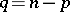is called its codimension. When considering foliations on a manifold with boundary one usually requires either transversality of the leaves to the boundary, or that a leaf which meets the boundary is completely contained within it. Complex-analytic foliations are defined in the obvious way. In what follows, it is, as a rule, assumed that foliations and mappings are differentiable, since this is the most important case.

The mapping (in the situation above)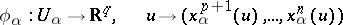is a submersion. The local leaves are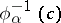,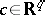. The system of local submersions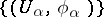is compatible (or coherent) in the sense that if, then nearone can pass fromto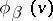by means of a certain local diffeomorphism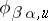(of class) of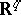, that is, for allsufficiently close toone has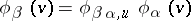. Conversely, ifis covered by domainsand one is given submersions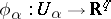that are compatible in the above sense, then by suitably "glueing together" theamong themselves, one obtains a foliation such that everyis contained in some leaf.

By assigning to every point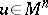the tangent space to the leaf passing through this point one obtains a field of-dimensional tangent subspaces (in other words, a-dimensional distribution), called the tangent field of the foliation. When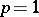, any field of-dimensional tangent subspaces, under the most minimal requirements of differentiability, is the tangent field of a uniquely determined foliation. When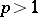this is not so. This question is of a local nature (see Frobenius theorem). The direct application of Frobenius' theorem to an involutive distribution shows that, when the corresponding conditions hold, there is a system of compatible local submersions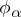whose tangent field is tangent to, passage to a foliation being realized by means of appropriate "glueing together" (for an alternative, more detailed, description of this see ).

The notion of a foliation evolved in the 1940s in a series of papers of G. Reeb and Ch. Ehresmann, culminating in the book  (for its history, see ), and was concerned with passing to the global point of view. This was stimulated in part by the theory of smooth dynamical systems (cf. Dynamical system), where the decomposition of the phase manifold (with the exception of the equilibrium positions, cf. Equilibrium position) into trajectories is a one-dimensional foliation. Flows on surfaces occupy a special place in this theory (see Poincaré–Bendixson theory; Differential equations on a torus; Kneser theorem), in which the trajectories locally partition the space; this helped to focus attention on foliations of codimension 1. Another example of a foliation, also studied in the 1940s, is the decomposition of a Lie group into cosets by an analytic subgroup (not necessarily closed) (see ). Finally, in the complex domain, the solutions of a differential equation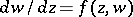with analytic right-hand side form (from a real point of view) a two-dimensional foliation.

After this early work there was a gap in the development of the theory of foliations, which still lacked significant results. Intensive development began with the work of A. Haefliger  and S.P. Novikov , the most well-known results of which are as follows (see ): A foliation of codimension 1 on a three-dimensional sphere has a compact leaf  and cannot be analytic , although Reeb constructed a foliation of class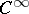. At that time, in the study of certain dynamical systems (-systems, cf.-system, and others related to them), there arose certain auxiliary foliations (not of codimension 1) which also stimulated the study of foliations (see , ). All these papers and a series of later ones were concerned with the "geometric" or "qualitative" aspect of the theory . In this much attention was paid to foliations of codimension 1, the existence of compact leaves, stability theorems (establishing that, under certain conditions, foliations with a compact leaf are structured like fibrations, in a neighbourhood of the leaf or globally; the first theorems of this type were already proved by Reeb, see ), the "growth" characteristic of the leaves (that is, the dependence onof the-dimensional volume of a geodesic sphere of radiuson a leaf), and their fundamental groups. Consider the following, recently solved, question: If a closed manifoldhas a-dimensional foliation all leaves of which are compact, then is the-dimensional volume of the leaves necessarily bounded? D. Epstein, D. Sullivan and others have shown that the answer is positive only when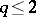(see ).

There later arose a "homotopy" direction of the theory, whose prototype was the homotopy theory of fibre bundles (cf. Fibre space). One of the difficulties here stemmed from the fact that for foliations there is in general no analogue of the induced fibre bundle. This necessitates the study of more general objects called Haefliger structures (cf. Haefliger structure) (a kind of foliation with singularities), for which such an analogue exists.

Two foliations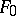and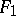onare called concordant if there is a foliation on the "cylinder"(having the same codimension) whose leaves are transversal to the "floor" and "roof" of the cylinder and "cut out" on them the foliationsand, respectively. Concordance of Haefliger structures is defined in a similar way. Every Haefliger structure is concordant to one which, outside a set of "singular points" on, corresponds to some foliation, and certain conditions hold for the behaviour of the leaves of the latter around these points. In this sense, a Haefliger structure can be thought of as a foliation with singularities. There is a natural bijection between the classes of concordant Haefliger structures and the homotopy classes of continuous mappings ofinto a so-called classifying space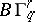(wheredenotes the codimension andthe smoothness class of the Haefliger structure).

Homotopy theory establishes which homotopy objects determine concordance of foliations: two foliations are concordant if and only if they are concordant as Haefliger structures and their tangent fields are homotopic (see , , ). A related result is the proof of the existence of-dimensional foliations on all open(see ) and on those closedon which there is a continuous field of-dimensional tangent subspaces (which is an obvious necessary condition, see , ). Several authors had earlier proved existence theorems for foliations on various manifolds by direct construction . The idea (see , ) is to begin with a foliation with singularities, and then liquidate them by modifying the foliation in a certain way. The case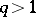is relatively simple (see , ) and the liquidation of singularities can be carried out in the spirit of the "geometric" theory ; the case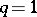is more complicated .

A mapping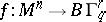induces a mapping of cohomology groups that leads to the concept of a characteristic class of a foliation. The "homological" or "quantitative" theory of foliations arising from this (see , , ) also includes certain results obtained earlier without reference to, such as the Godbillon–Weil invariant (for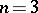see ), or R. Bott's necessary conditions for a continuous field of tangent subspaces to be homotopic to the tangent field of a foliation.